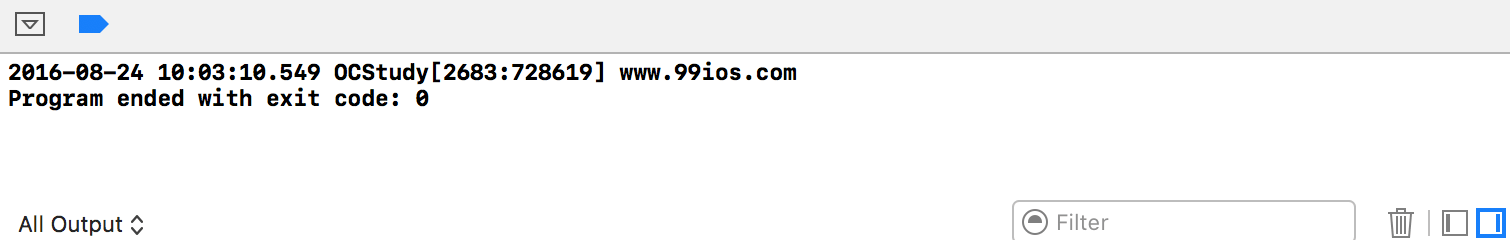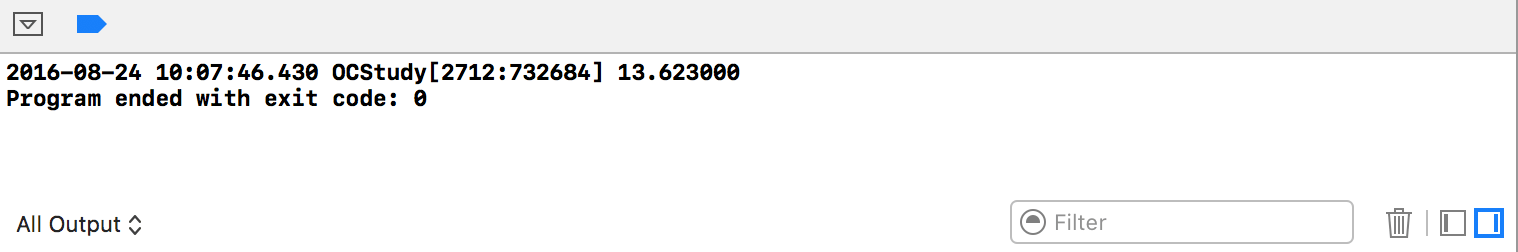# 无参宏

`````` # define 宏名 宏体
``````

``````// 定义了一个符号常量，用PI这个符号代表常量3.14，在后面代码中要用到3.14的时候都可以用PI代替
#define PI 3.14
// 定义了一个符号常量，用LARGE代表（100 + 100），宏体中常量多于一个时需要用一对()括起来
#define LARGE (100 + 100)
// 定义了一个符号常量，用WEBNAME代表了一个字符串"www.99ios.com"
#define WEBNAME "www.99ios.com"
// 定义了一个符号常量，用AND代表了 && 符号
#define AND &&
// 在宏定义中，可以使用另一个宏定义的值
#define TWO_PI (2.0 * PI)
``````

# 有参宏

`````` #define 宏名（参数列表） 宏体
``````

``````// 定义一个有一个参数的宏，求参数的平方值
#define SQUARE(a)    ((a) * (a))  // 宏体中所有的参数必须用一对()括起来
// \ 被称为续行符，表示下一行是本行的延续。在 \ 符号所在行之后不能加任何空白字符
// 定义一个有两个参数的宏，求两个参数中较大的值，宏体可以是某些代码段，用{}括起来
#define LARGER(a, b)  ({ \
int m = a, n = b; m > n ? m : n; \
})
// 上面的代码与下方代码等价
#define LARGER(a, b) ({int m = a, n = b; m > n ? m :n;})
``````

``````#define SQUARE(a)    ((a) * (a))
``````
``````int a = 3, b = 4;
NSLog(@"square = %d\n", SQUARE(a + b)); // ((3 + 4) * (3 + 4))
``````

(3 + 4 * 3 + 4)

# 运算符"#"

``````#define STRING(n) #n
``````
``````NSLog(@"%s", STRING(www.99ios.com));
``````# 运算符"##"

##运算符用于把参数连接到一起。预处理程序把出现在##两侧的参数合并成一个符号。

``````#define CONNECT(a, b, c) (a##b##c)
``````
``````NSLog(@"%f", CONNECT(13.6, 2, 3));
``````# 示例代码

https://github.com/99ios/6.3.1